## Hyperfine Structure

The interaction between the spin of the nucleus and the angular momentum of the electron causes a further (hyperfine) splitting of atomic states. It is called hyperfine because it is also order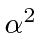like the fine structure corrections, but it is smaller by a factor of aboutbecause of the mass dependence of the spin magnetic moment for particles.

The magnetic moment of the nucleus iswhere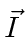is the nuclear spin vector. Because the nucleus, the proton, and the neutron have internal structure, the nuclear gyromagnetic ratio is not just 2. For the proton, it is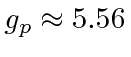.

We computed the hyperfine contribution to the Hamiltonian forstates.Now, just as in the case of the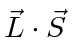, spin-orbit interaction, we will define the total angular momentumIt is in the states of definiteandthat the hyperfine perturbation will be diagonal. In essence, we are doing degenerate state perturbation theory. We could diagonalize the 4 by 4 matrix for the perturbation to solve the problem or we can use what we know to pick the right states to start with. Again like the spin orbit interaction, the total angular momentum states will be the right states because we can write the perturbation in terms of quantum numbers of those states.For the hydrogen ground state we are just adding two spinparticles so the possible values are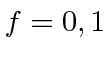. The transition between the two states gives rise to EM waves with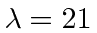cm.

We will work out the effect of an external B field on the Hydrogen hyperfine statesboth in the strong field and in the weak field approximation. We also work the problem without a field strength approximation. The always applicable intermediate field strength result is that the four states have energies which depend on the strength of the B field. Two of the energy eigenstates mix in a way that also depends on B. The four energies are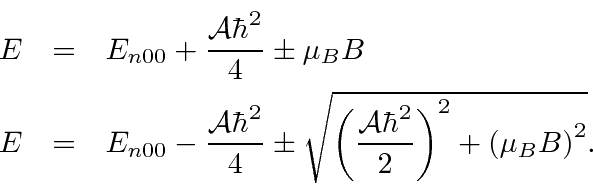Jim Branson 2013-04-22